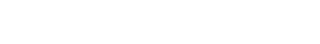Between watts, watt hours, energy, and power, it can become confusing to work out what each term means. Although watts and watt-hours are related terms, they are not the same thing. In this article, we’ll help you understand the difference.

## What is a watt?

A watt is a unit of power. It is the amount of energy an item needs to function; the rate at which energy is consumed.
One watt is equivalent to electricity flowing at a rate of one joule (unit of energy) per second.

## What is power?

Power is the rate at which energy is either produced or consumed; the rate at which energy flows. Power is measured in watts.

## What is energy?

Energy is the capacity to do work – such as creating light, heat, or motion.

## What is a watt-hour?

A watt-hour is a unit of energy. It is the amount of energy an item consumes over a given timeframe; a way to measure the amount of work generated or performed.
Watt hours are generally measured in kilowatt-hours.

## What’s the difference between watts and watt-hours?

• Watts measure power
• Watt-hours measure energy

## What is a watt? A helpful analogy

You can think of watts and watt-hours as speed and distance.

• Speed = how fast you drive at an instant in time (like a how much power is used at an instant in time; a watt)
• Distance = the length, or amount that you drive over a period of time (like how long energy is used for; a watt-hour)

## What is a kilowatt-hour?

A kilowatt-hour is a measure of electrical energy equivalent to a power consumption of one thousand watts for one hour.
I.e. 1 kWh = 1000 Wh

### Fun facts about the kilowatt

• One kilowatt is equal to approximately 1.34 horsepower
• A surface area of one square meter on Earth (close to the equator) receives around one kilowatt of sunlight from the sun on a clear day

## What is a megawatt?

A megawatt (MW) is one million watts. This measure of energy is used for high energy consumers such as:

• large electric motors
• aircraft carriers
• Submarines
• server farms
• data centres
• scientific research equipment

## Variations on kilowatt hours

• 1 kilowatt hour (kWh) = 1,000 watt hours (Wh)
• 1 megawatt hour (MWh) = 1,000 kilowatt hours (kWh)
• 1 gigawatt hour (GWh) = 1,000 megawatt hours (MWh)

## What is a watt? An example

A 60-watt lightbulb will consume electricity at a rate of 60 watts.

If you keep this light on for an hour, you will have used 60 watt-hours – 0.06 kilowatt-hours. (Remember, 1000 watts = 1 kilowatt.)

This means that it takes 0.06 kWh of energy to run a lightbulb for an hour.

The more powerful an appliance, the higher the watts.

## How do I know an appliance’s wattage?

Information about an appliance’s energy needs appears on its technical description.

## What unit do I see on my electricity bill?

Electricity suppliers measure the electricity you’ve used in a given time period (usually a month) in kilowatt -hours (kWh).

## How are energy costs calculated?

Your supplier will charge you a unit cost per kilowatt-hour. They will take the amount of kWh used in a month multiplied by the unit cost to give you your energy consumption costs for that month. This unit charge can change according to demand and other charges.

## Where did the watt get its name?

The watt is named after a Scottish inventor named James Watt, inventor of the steam engine. The name for a unit of energy was initially proposed by C. William Siemens in August 1882 in his President’s Address to the Fifty-Second Congress of the British Association for the Advancement of Science. He proposed that Watt would be an appropriate name for a unit of power.

Knowing the difference between watts and watt-hours can help you better understand your electricity bill and energy consumption.